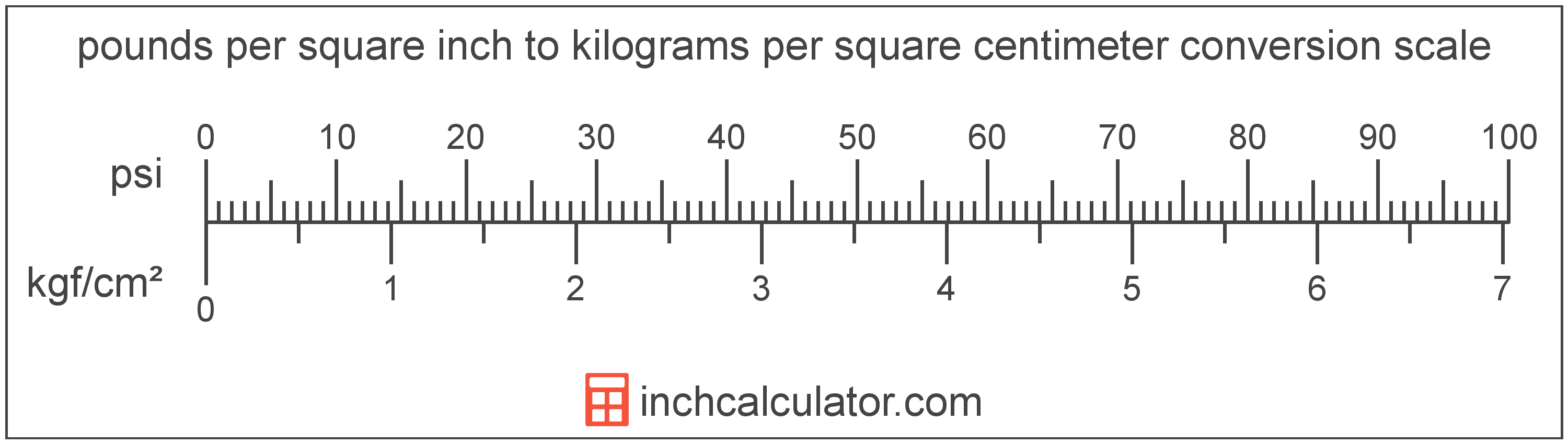# Pounds per Square Inch to Kilograms per Square Centimeter Converter

Enter the pressure in pounds per square inch below to get the value converted to kilograms per square centimeter.

Results in Kilograms per Square Centimeter:1 psi = 0.070307 kgf/cm²

## How to Convert Pounds per Square Inch to Kilograms per Square Centimeter

To convert a measurement in pounds per square inch to a measurement in kilograms per square centimeter, multiply the pressure by the following conversion ratio: 0.070307 kilograms per square centimeter/pound per square inch.

Since one pound per square inch is equal to 0.070307 kilograms per square centimeter, you can use this simple formula to convert:

kilograms per square centimeter = pounds per square inch × 0.070307

The pressure in kilograms per square centimeter is equal to the pressure in pounds per square inch multiplied by 0.070307.

For example, here's how to convert 5 pounds per square inch to kilograms per square centimeter using the formula above.
kilograms per square centimeter = (5 psi × 0.070307) = 0.351534 kgf/cm²Pounds per square inch and kilograms per square centimeter are both units used to measure pressure. Keep reading to learn more about each unit of measure.

## What Are Pounds per Square Inch?

One pound per square inch is the pressure of equal to one pound-force per square inch.

The pound per square inch is a US customary and imperial unit of pressure. A pound per square inch is sometimes also referred to as a pound-force per square inch. Pounds per square inch can be abbreviated as psi; for example, 1 pound per square inch can be written as 1 psi.

PSI can be expressed using the formula:
1 psi = 1 lbf / in2

Pressure in pounds per square inch are equal to the pound-force divided by the area in square inches.

## What Are Kilograms per Square Centimeter?

One kilogram per square centimeter is the pressure of equal to one kilogram-force per square centimeter.

The kilogram per square centimeter is a non-SI metric unit for pressure. A kilogram per square centimeter is sometimes also referred to as a kilogram per square centimetre or kilogram-force per centimeter square. Kilograms per square centimeter can be abbreviated as kgf/cm²; for example, 1 kilogram per square centimeter can be written as 1 kgf/cm².

In the expressions of units, the slash, or solidus (/), is used to express a change in one or more units relative to a change in one or more other units. For example, kgf/cm² is expressing a change in weight relative to a change in area.

The unit is deprecated and not permitted for use with SI units, although it is still in use as some precision measuring devices still use the unit.

Kilograms per square centimeter can be expressed using the formula:
1 kgf/cm2 = 1 kgf / cm2

Pressure in kilograms per square centimeter are equal to the kilogram-force divided by the area in square centimeters.

## Pound per Square Inch to Kilogram per Square Centimeter Conversion Table

Table showing various pound per square inch measurements converted to kilograms per square centimeter.
Pounds Per Square Inch Kilograms Per Square Centimeter
1 psi 0.070307 kgf/cm²
2 psi 0.140614 kgf/cm²
3 psi 0.210921 kgf/cm²
4 psi 0.281228 kgf/cm²
5 psi 0.351534 kgf/cm²
6 psi 0.421841 kgf/cm²
7 psi 0.492148 kgf/cm²
8 psi 0.562455 kgf/cm²
9 psi 0.632762 kgf/cm²
10 psi 0.703069 kgf/cm²
11 psi 0.773376 kgf/cm²
12 psi 0.843683 kgf/cm²
13 psi 0.913989 kgf/cm²
14 psi 0.984296 kgf/cm²
15 psi 1.0546 kgf/cm²
16 psi 1.1249 kgf/cm²
17 psi 1.1952 kgf/cm²
18 psi 1.2655 kgf/cm²
19 psi 1.3358 kgf/cm²
20 psi 1.4061 kgf/cm²
21 psi 1.4764 kgf/cm²
22 psi 1.5468 kgf/cm²
23 psi 1.6171 kgf/cm²
24 psi 1.6874 kgf/cm²
25 psi 1.7577 kgf/cm²
26 psi 1.828 kgf/cm²
27 psi 1.8983 kgf/cm²
28 psi 1.9686 kgf/cm²
29 psi 2.0389 kgf/cm²
30 psi 2.1092 kgf/cm²
31 psi 2.1795 kgf/cm²
32 psi 2.2498 kgf/cm²
33 psi 2.3201 kgf/cm²
34 psi 2.3904 kgf/cm²
35 psi 2.4607 kgf/cm²
36 psi 2.531 kgf/cm²
37 psi 2.6014 kgf/cm²
38 psi 2.6717 kgf/cm²
39 psi 2.742 kgf/cm²
40 psi 2.8123 kgf/cm²

## References

1. National Institute of Standards and Technology, NIST Guide to the SI, Chapter 6: Rules and Style Conventions for Printing and Using Units, https://www.nist.gov/pml/special-publication-811/nist-guide-si-chapter-6-rules-and-style-conventions-printing-and-using